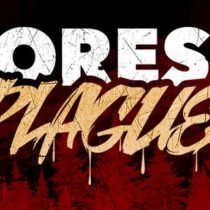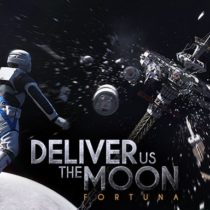#### Simulation GAMES• Simulation
• Casual
• Visual Novel• Action
• Simulation
• Horror
• Survival• Simulation
• Building
• Racing
• Open World
• Management
• Strategy• Strategy
• Simulation
• RPG
• Turn-Based
• Sci-fi
• Anime• Simulation
• Management
• Strategy• Simulation
• Action
• Building
• Sci-fi
• Shooter
• Strategy• Action
• Simulation
• RPG
• Strategy
• Anime• Simulation
• Puzzle
• Casual
• Strategy• Simulation
• Casual
• RPG
• Strategy
• Anime
• Management• Simulation
• Casual
• RPG
• Anime• Casual
• Simulation
• Strategy
• Nudity• Action
• Casual
• Simulation
• Strategy
• Horror